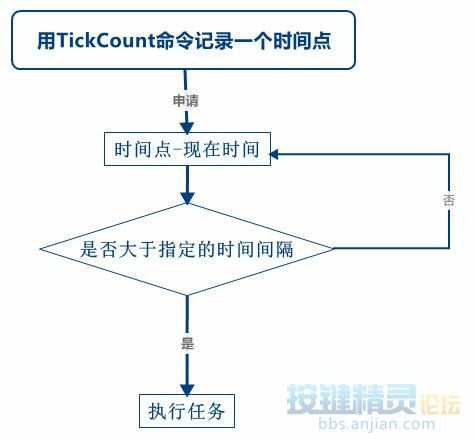1#【按键学院】安卓课程(115768679)

 现在手游上很多活动、任务什么的都是在固定的时间或者间隔一段时间之后才能继续做的。那如何用代码来实现定时的功能就和脚本是否有效率挂上了勾了。只有当时间到的时候才去执行任务，这样的脚本就比一直循环去查看任务有效率多了。这篇院刊就给大家带来几种实现定时的方法。

 第一种方法1. Dim t=TickCount()

2. Do
3. If TickCount() - t >10000 Then
4. TracePrint "时间到了，开始执行任务"
5. Else
6. TracePrint "继续等待"
7. End If
8. Delay 1000
9. Loop

1. Dim TimeID()

2. Function RemTime(id)
3. TimeID(id) = TickCount()
4. RemTime = TimeID(id)
5. End Function

6. Function TimeDiff(id, t)
7. Dim t1 = TickCount()
8. //计算时间差
9. If t1 - TimeID(id) >= t Then
10. TimeDiff = true
11. //把该记号的时间重置成当前时间，以便可以再次计时
12. TimeID(id) = t1
13. Else
14. TimeDiff = false
15. End If
16. End Function

1. Call RemTime(1)
2. Call RemTime(2)

3. Do
4. If TimeDiff(1, 10000) Then
5. Call 任务1
6. End If
7. If TimeDiff(1, 30000) Then
8. Call 任务2
9. End If
10. Loop

 第二种方法

1. TracePrint DateTime.Format("%H:%S")

1. Function Timing_time(t)
2. Dim t1 = DateTime.Format("%H:%S")
3. If t = t1 Then
4. Timing = true
5. Else
6. Timing = false
7. End If
8. End Function

9. If Timing_time("20:30") Then
10. TracePrint "现在已经20:30了，可以执行任务"
11. Else
12. TracePrint "时间还没到"
13. End If

1. Function Timing_date(t)
2. Dim t1 = DateTime.Format("%Y-%m-%d")
3. If t = t1 Then
4. Timing = true
5. Else
6. Timing = false
7. End If
8. End Function

9. If Timing_date("2016-03-05") Then
10. TracePrint "现在已经2016-03-05了，可以执行任务"
11. Else
12. TracePrint "时间还没到"
13. End If